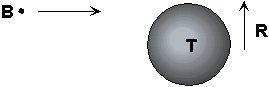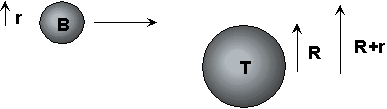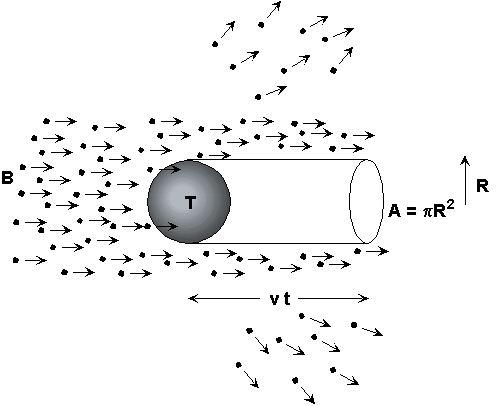## What is a Cross Section?

When a beam of particles of type B strikes a target consisting of particles of type T, some of the B particles pass directly through the target while others are deflected. Those that are deflected are said to interact or collide with the T particles. The cross section, which is denoted by s by physicists, is a measure of the effectiveness of the B-T interaction. The larger the cross section, the more likely it is that the B particles are deflected. A cross section depends on the types of particles involved and usually depends on the energy of the particles in the beam. To emphasize the dependence on particle types, we denote the cross section by sBT below.

If one throw darks at a board of area A then the chance of hitting the dark board is proportional to A. Indeed, the cross section for this process is A. Cross section has dimensions of area or length2.

In general, the cross section is the effective area of the collision region. Here is another classical example that illustrates the concept. Suppose that the target T is a solid ball of radius R and suppose that the beam particles B are point-like. A B particle will strike the ball if it passes within a distance of R of the center of the ball. The cross section sBT for this case is the area of a circle of radius R or p R2.The cross section for point-like particles colliding with a sphere
is just the area of the sphere projected onto
the transverse plane, that is, a circular disk of radius R.

If the beam particles B are also balls of radius r, then a B is deflected if it comes within a distance of R+r of the center of the ball T. The cross section s BT for this case is p (R+r)2.The cross section for sphere-sphere collisions is
the area of a circular disk of radius R+r.

In the microscopic world, elementary particles cannot be considered balls and they do not interact so mechanically. They can interact at a distance – similar to the way two magnets affect each other without touching one another. Furthermore, physicists cannot simply examine the particles to determine the cross section unlike the case of macroscopic balls, whose radii are readily measured [However, if the interactions are precisely known, theorists can calculate the expected cross section]. Basically, experimentalists smash a beam of particles against a target (or another beam) and observe the results. From this information, the cross section can be computed.

To see how this works, return to the macroscopic, mechanical example of a beam of pointlike B particles impinging on target balls T of radii R. Suppose that the density of beam particles is nbeam (nbeam is the number of B particles per unit volume) and suppose that the beam particles are travelling with a speed v. Recall that the number of collisions is number of B particles that come within a distance of R of the center of a target ball. For the moment, assume that there is only one T particle. The ball T casts a "shadow" in the shape of a cylinder in the beam: the region behind T is devoid of particles.The particles that have been scattered would have been located
in the cylinder had the sphere not been there.

The number Ninteractions of particles that have collided is the number of B's that would be in the "shadow" region had T not been present. This number is the volume of the shadow region V times the beam density nbeam:

Ninteractions = nbeam V

The volume V of the cylinder is the cross sectional area p R2 times the length L of the cylinder. Let t be the time during which the beam particles B have been striking the target. Since, in a time t, the beam particles travel a distance of vt, L = vt. Hence, V = p R2 vt. Substituting this result for V into the above equation for the number of interactions leads to Ninteractions = n p R2 vt. The number Ninteractions_per_unit_time of reactions per unit time is Ninteractions_per_unit_time = Ninteractions /t or

Ninteractions_per_unit_time = nbeam p R2 v

Solving for pR2, which is the cross section for this particular case, one finds

s BT = Ninteractions_per_unit_time / Jbeam

where Jbeam is known as the beam flux and is equal to nbeam v. This equation defines the cross section in general.

Usually the target does not consist of a single particle. If Ntarget is the number of particles present in the target then the number of collisions per unit time is Ntarget times greater than the case of a single particle, and one must divide by this factor if the cross section is to represent the effective area of interaction between a single beam particle and a single target particle:

s BT = Ninteractions_per_unit_time / (Jbeam Ntarget)     (basic cross section formula)

The number of target particles Ntarget is often computed using Ntarget = ntarget Vtarget, where Vtarget is the volume of the target that is in the line of the beam and ntarget is the number density of the target particles, that is, the number of T particles per unit volume. This equation reproduces the correct result for the cross section in the classical case where B and T are balls and defines the cross section for the general situation.

Thus, the procedure for computing the cross section s BT in any experiment for which a beam of particles is directed at a target is as follows:
(1) Measure the number of collisions per unit time: Ninteractions_per_unit_time
(2) Determine the number of target particles Ntarget, perhaps by taking the number density of T particles times the volume of the target.
(3) Determine the beam flux Jbeam by multiplying the number density of the beam times the speed of a beam particle.
(4) Use the basic cross section formula given above: Take the quantity in (1) (Ninteractions_per_unit_time) and divide it by the quantities in (2) (Ntarget) and (3) (Jbeam).

This report was prepared by the staff of Jupiter Scientific, an organization devoted to the promotion of science through books, the internet and other means of communication.

This web page may NOT be copied onto other web sites, but other sites may link to this page.